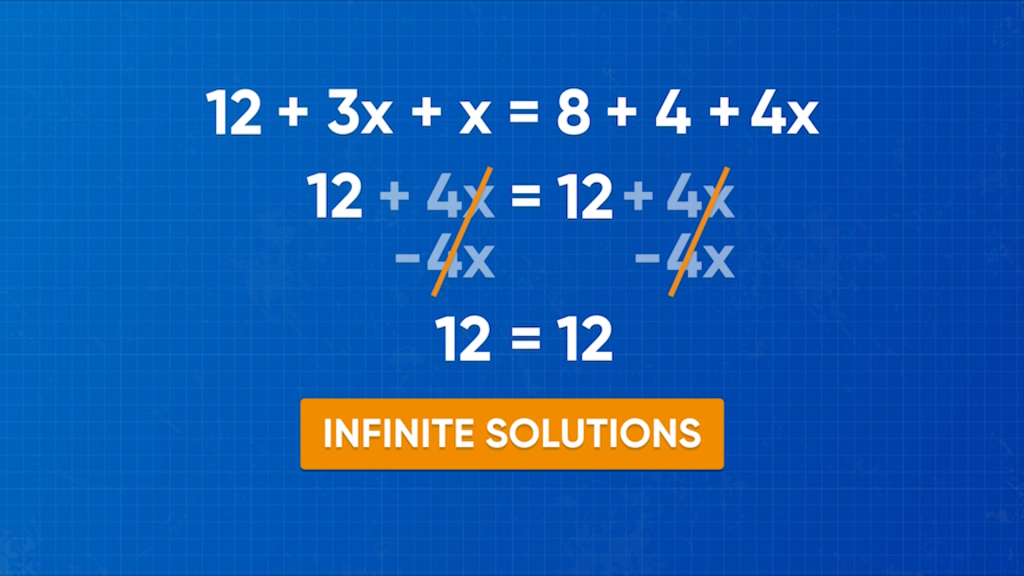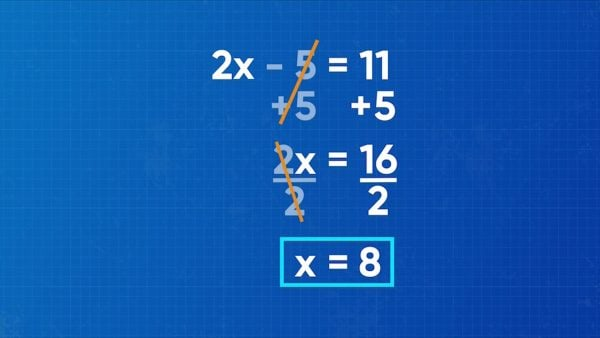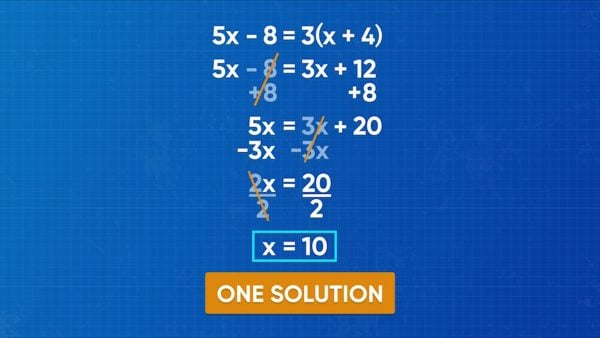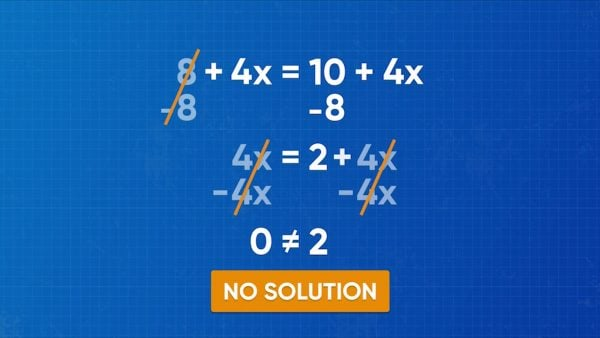Determine Number of Solutions for Linear Equations Video - Grades 6-8
1%
It was processed successfully!HOW MANY SOLUTIONS DOES AN ALGEBRAIC EQUATION HAVE?

Algebraic equations can have 1 solution. They may also have infinitely many solutions, which means that any value of the variable makes the equation true. They may also have no solutions, which means that no value of the variable makes the equation true.

To better understand how to determine the number of solutions for linear equations …

HOW MANY SOLUTIONS DOES AN ALGEBRAIC EQUATION HAVE?. Algebraic equations can have 1 solution. They may also have infinitely many solutions, which means that any value of the variable makes the equation true. They may also have no solutions, which means that no value of the variable makes the equation true. To better understand how to determine the number of solutions for linear equations …

## LET’S BREAK IT DOWN!

### Review how to solve an equation.Emily wants to solve the equation 2x-5=11. She knows she needs to do two steps to solve and use inverse operations to isolate the x. First, 5 is subtracted on the left side, so add 5 to both sides of the equation. 2x-5+5=11+5. This simplifies to 2x=16. Next, the x is multiplied by 2, so divide both sides by 2. [ggfrac]2x/2[/ggfrac] = [ggfrac]16/2[/ggfrac]. This simplifies to x=8. x has been isolated, so you know that x is equal to 8. Try this yourself: Solve for x in the equation 3x+5=17.

Review how to solve an equation. Emily wants to solve the equation 2x-5=11. She knows she needs to do two steps to solve and use inverse operations to isolate the x. First, 5 is subtracted on the left side, so add 5 to both sides of the equation. 2x-5+5=11+5. This simplifies to 2x=16. Next, the x is multiplied by 2, so divide both sides by 2. [ggfrac]2x/2[/ggfrac] = [ggfrac]16/2[/ggfrac]. This simplifies to x=8. x has been isolated, so you know that x is equal to 8. Try this yourself: Solve for x in the equation 3x+5=17.

### Equations can have one solution.Emily wants to solve the equation 5x-8=3(x+4). This equation takes multiple steps to solve and has variables on both sides of the equation! On the right side, the 3 is multiplied by an expression in brackets. To simplify the right side, you need to use the distributive property. Multiply 3 by every term inside the brackets. 3(x + 4) = 3(x) + 3(4) = 3x + 12. Now the equation can be written as 5x-8=3x+12. Isolate the x-terms on one side of the equation (it doesn’t matter which side). Emily decides to put the x-terms on the left side, so she focuses on eliminating the -8 first. Add 8 to both sides. 5x-8+8=3x+12+8, which simplifies to 5x=3x+20. Next, subtract 3x from both sides. 5x-3x=3x+20-3x, which simplifies to 2x=20. The last step to isolate the x is to divide both sides by 2. [ggfrac]2x/2[/ggfrac] = [ggfrac]20/2[/ggfrac]. Then x = 10. The equation has one solution, and that solution is 10. Try this yourself: Solve for x in 8x-12=-4(x+6).

Equations can have one solution. Emily wants to solve the equation 5x-8=3(x+4). This equation takes multiple steps to solve and has variables on both sides of the equation! On the right side, the 3 is multiplied by an expression in brackets. To simplify the right side, you need to use the distributive property. Multiply 3 by every term inside the brackets. 3(x + 4) = 3(x) + 3(4) = 3x + 12. Now the equation can be written as 5x-8=3x+12. Isolate the x-terms on one side of the equation (it doesn’t matter which side). Emily decides to put the x-terms on the left side, so she focuses on eliminating the -8 first. Add 8 to both sides. 5x-8+8=3x+12+8, which simplifies to 5x=3x+20. Next, subtract 3x from both sides. 5x-3x=3x+20-3x, which simplifies to 2x=20. The last step to isolate the x is to divide both sides by 2. [ggfrac]2x/2[/ggfrac] = [ggfrac]20/2[/ggfrac]. Then x = 10. The equation has one solution, and that solution is 10. Try this yourself: Solve for x in 8x-12=-4(x+6).

### Equations can have no solutions.Adesina is solving 4x+8=4x+10. There is a variable on both sides of the equal sign, so move the variables to one side and the constants to the other. It doesn’t matter which you do first. First, let's remove the + 8 from the left side by subtracting 8 from both sides. 4x+8-8=4x+10-8, which simplifies to 4x=4x+2. Next, remove the 4x from the right side by subtracting 4x from both sides. 4x-4x=4x+2-4x, or 0=2. This equation is not true! It does not matter what value of x you choose, you cannot make the left and right sides of the equation equal. This means that the equation has no solutions. Try this yourself: Show algebraically that 2x+4=2x+8 has no solutions.

Equations can have no solutions. Adesina is solving 4x+8=4x+10. There is a variable on both sides of the equal sign, so move the variables to one side and the constants to the other. It doesn’t matter which you do first. First, let's remove the + 8 from the left side by subtracting 8 from both sides. 4x+8-8=4x+10-8, which simplifies to 4x=4x+2. Next, remove the 4x from the right side by subtracting 4x from both sides. 4x-4x=4x+2-4x, or 0=2. This equation is not true! It does not matter what value of x you choose, you cannot make the left and right sides of the equation equal. This means that the equation has no solutions. Try this yourself: Show algebraically that 2x+4=2x+8 has no solutions.

### Equations can have infinitely many solutions.Emily is solving 12+3x=3x+12. Let's start by removing the 12 from the left side. So, subtract 12 from both sides: 12+3x-12=3x+12-12. When simplified this becomes 3x=3x. Next, remove the 3x from the right side by subtracting 3x from both sides. 3x-3x=3x-3x, which simplifies to 0=0. This statement is true. It doesn’t matter what value of x you choose, the left side is equal to the right side. This means that there are infinitely many solutions. Try this yourself: Show algebraically that 4+10x=2(5x+2) has infinitely many solutions.

Equations can have infinitely many solutions. Emily is solving 12+3x=3x+12. Let's start by removing the 12 from the left side. So, subtract 12 from both sides: 12+3x-12=3x+12-12. When simplified this becomes 3x=3x. Next, remove the 3x from the right side by subtracting 3x from both sides. 3x-3x=3x-3x, which simplifies to 0=0. This statement is true. It doesn’t matter what value of x you choose, the left side is equal to the right side. This means that there are infinitely many solutions. Try this yourself: Show algebraically that 4+10x=2(5x+2) has infinitely many solutions.

## DETERMINING THE NUMBER OF SOLUTIONS FOR LINEAR EQUATIONS VOCABULARY

Algebraic equation
An equation with a variable in it.
True equation
An equation where the value of the left side equals the value of the right side.
False equation
An equation where the value of the left side does not equal the value of the right side.
Variable
A letter that represents an unknown number.
Inverse operations
Operations that are opposites, so one operation undoes the other operation. Addition and subtraction are inverses, and multiplication and division are inverses.
Solution to an equation
Value(s) for the variable(s) in an algebraic equation that make(s) the equation true.
When a term is multiplied by a set of brackets that contain an expression, each term in the expression must be multiplied by the term outside the brackets. a(b+c)=ab+ac.

## DETERMINING THE NUMBER OF SOLUTIONS FOR LINEAR EQUATIONS DISCUSSION QUESTIONS

### What is the distributive property?

The distributive property is a way of multiplying expressions that have more than one term in them. Every term in the first expression gets multiplied by every term in the second expression. For example, 3(x+4)=(3)(x)+(3)(4)=3x+12.

### When you solve an equation until there are no variables left, what are the possible solutions to the problem?

If there is one solution, one x is left in the equation. If the simplified equation has no variables left, then there are either no solutions or infinitely many solutions.

### How can you tell if an equation has infinitely many solutions?

If you simplify an equation so that there are no variables left, and the equation you are left with is true, then there are infinitely many solutions. It means no matter what x-value you substitute in, both sides of the equation are equal.

### How can you tell if an equation has no solutions?

If you simplify the equation so that there are no variables left, and the equation you are left with is untrue, then there are no solutions. It means that no matter what value of x you substitute in, both sides of the equation are never equal.

### How can you tell if an equation has one solution?

If you can see that there are a different number of x’s on each side of the equation, then the equation has only one solution, and you can find it using inverse operations. For example, in the equation 3(x+3)=2x+9, use distribution on the left side to get 3x+9=2x+9. Since there are 3 x’s on the left and 2 x’s on the right, there is only one solution.
X

## Success

We’ve sent you an email with instructions how to reset your password.
Ok